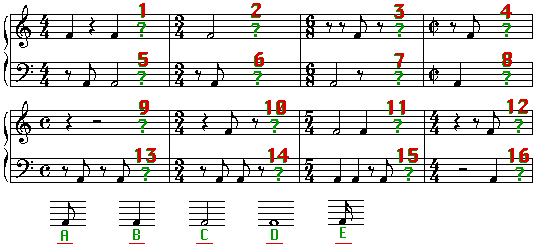Lesson 11 - Section 1
Lesson 11 - Section 1
In the following measures, choose the Note that is missing (A-E). You may want to write down your answers first and then enter them into the quiz below to get your score.Measure 1 is missing? (choose from notes A-E in the image above)
6 points
Measure 2 is missing?
6 points
Measure 3 is missing?
6 points
Measure 4 is missing?
6 points
Measure 5 is missing?
6 points
Measure 6 is missing?
6 points
Measure 7 is missing?
6 points
Measure 8 is missing?
6 points
Measure 9 is missing?
6 points
Measure 10 is missing?
6 points
Measure 11 is missing?
6 points
Measure 12 is missing?
6 points
Measure 13 is missing?
6 points
Measure 14 is missing?
6 points
Measure 15 is missing?
6 points
Measure 16 is missing?
10 points